## 2D Graphs

Cassiopeia includes a sophisticated 2D graph module for plotting functions. We will discuss a damped LC tank in this document and then present the result of the derivation in a 2D graph. If you are not interested in the concrete physical problem skip the math and scroll down to the graphs. :-) We consider the following electrical circuit consisting of a capacitance, a resistor and an inductance.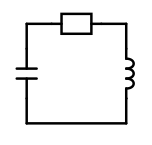This circuit is described mathematically by the following two differential equations:(1)(2)

The first equation can be rewritten as follows:We use the following approach to solve this linear differential equation: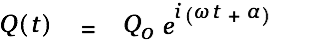The last equation is true for all t only if we assumeThis gets us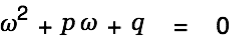We consider the first solution and insert the expression into our solution apporach.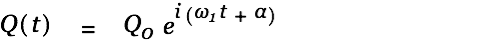We setand use Euler to rewriteThe differential equation is linear. Therefore both summands must be a solution for the differential equations.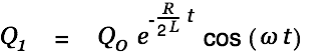A constant factor shouldn't harm a solution and the sum of two solutions is again a solution. We can thererefore writeThis can be transformed to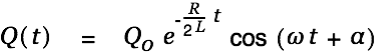withWe now use Eq. 2 to get an expression for the current in the circuit.We Alt-drag the expression for the angular frequency onto this last equation and replace Q with CU. This gets us the following two functions.Now choose SDM - FunctionGraph2D from the menu to insert a 2DGraph and drag the two equations onto the textview of the FunctionGraph2D inspector.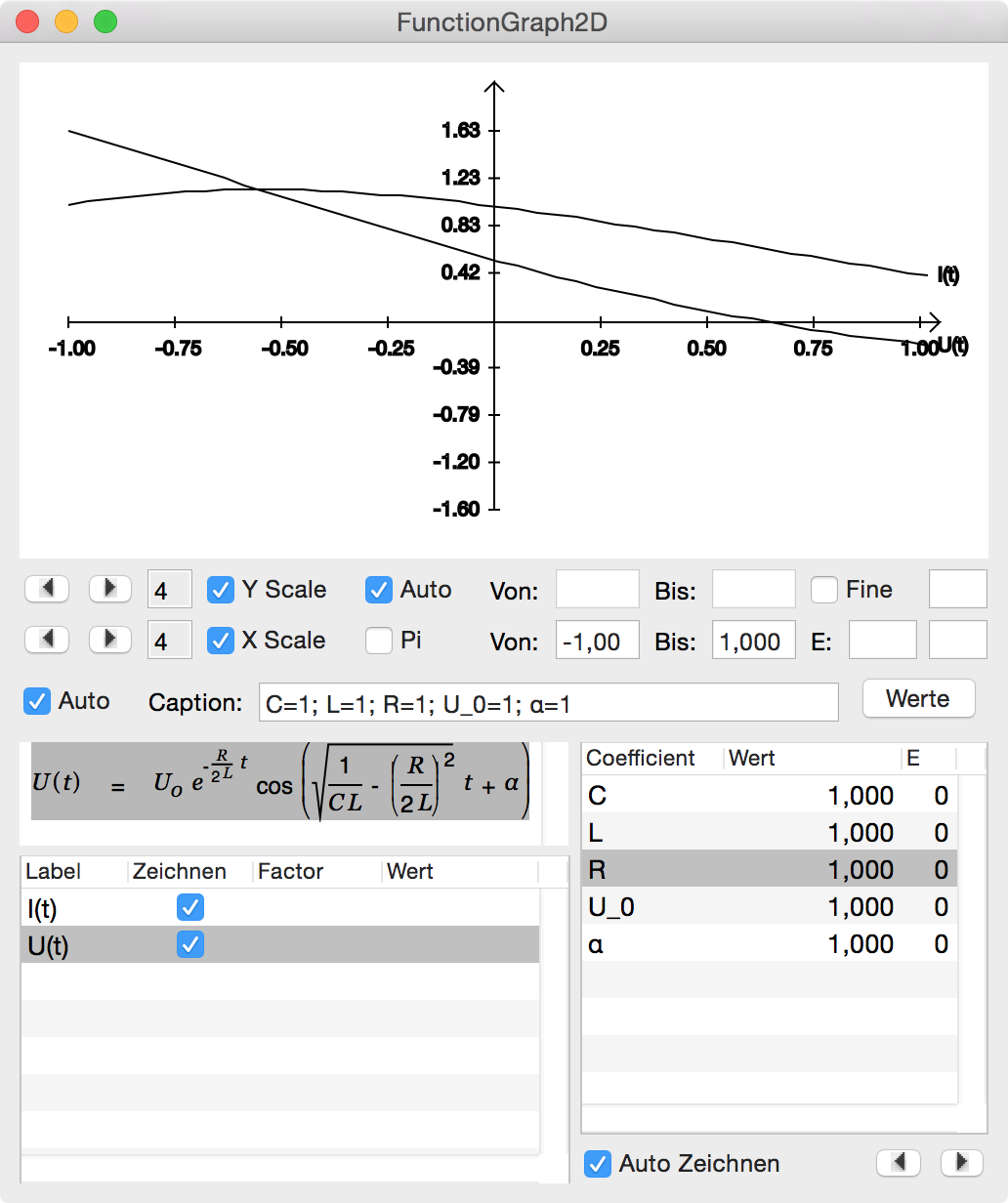The graph appears as follows In our document.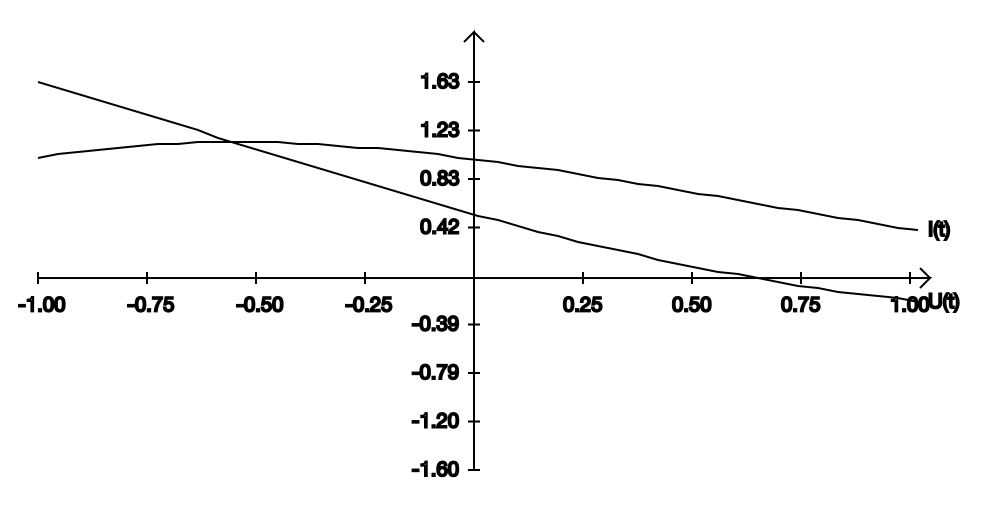We change the From: and To: values for the abscissa to 0 - 100 ms. After modifying the values press <Return> in one of the fields to trigger redraw of the graph.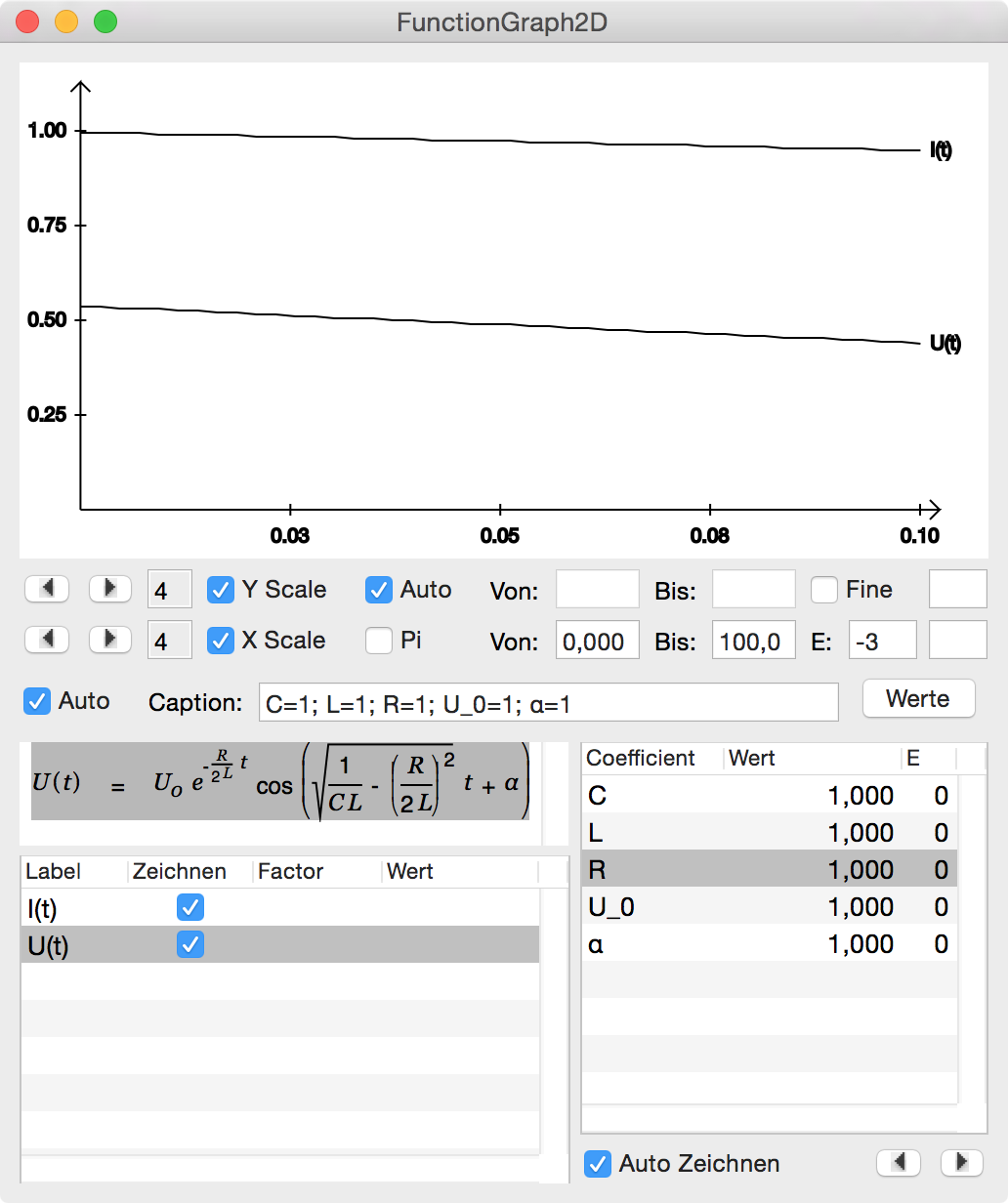Now change the coefficients C, L and R to more realistic values. Also check the Fine box to get a more accurate rendering of the graph.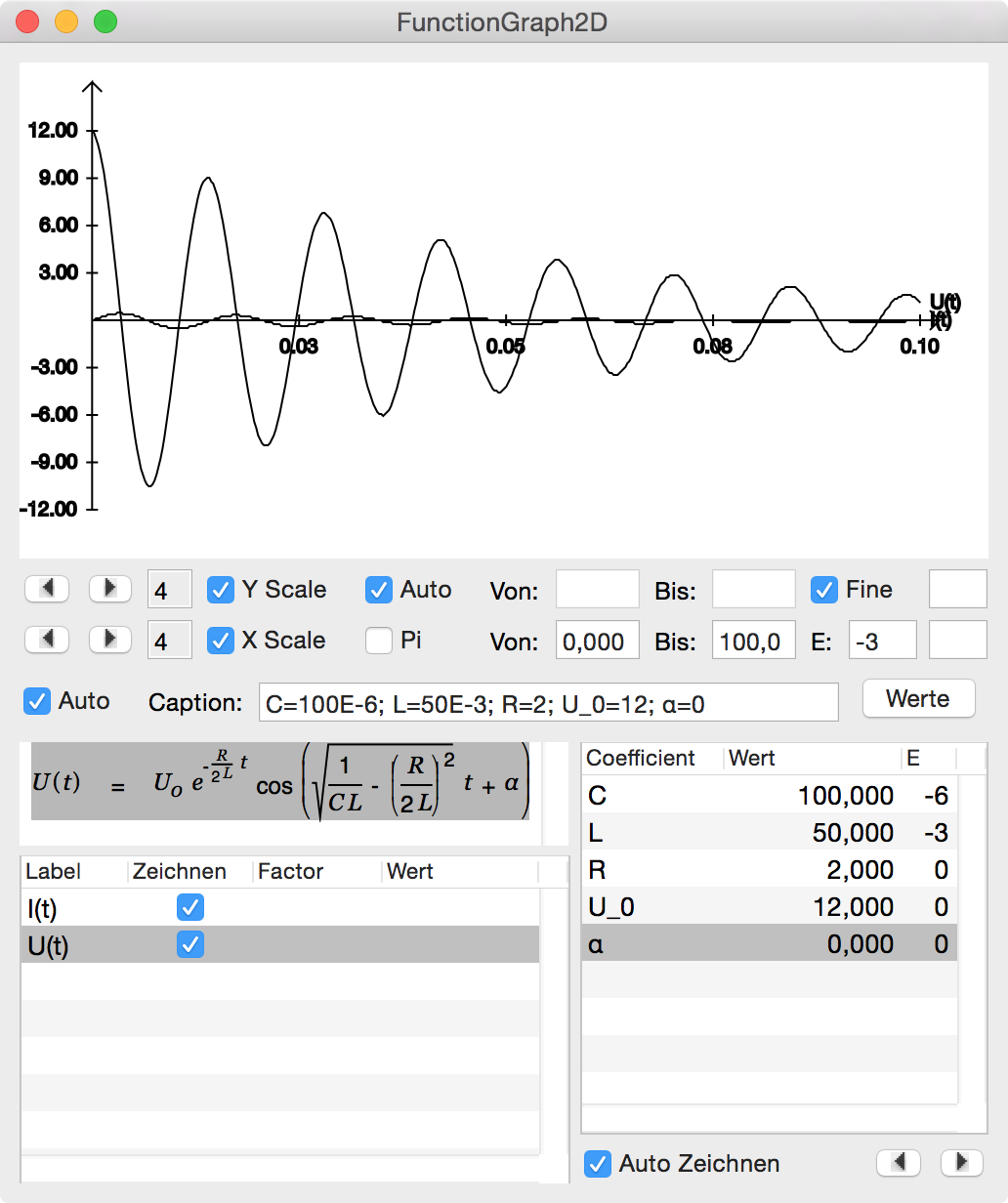Choose Tools - Colors from the menu and drag red color onto the current function in the tablevie win the lower left and blue color onto the function for the voltage.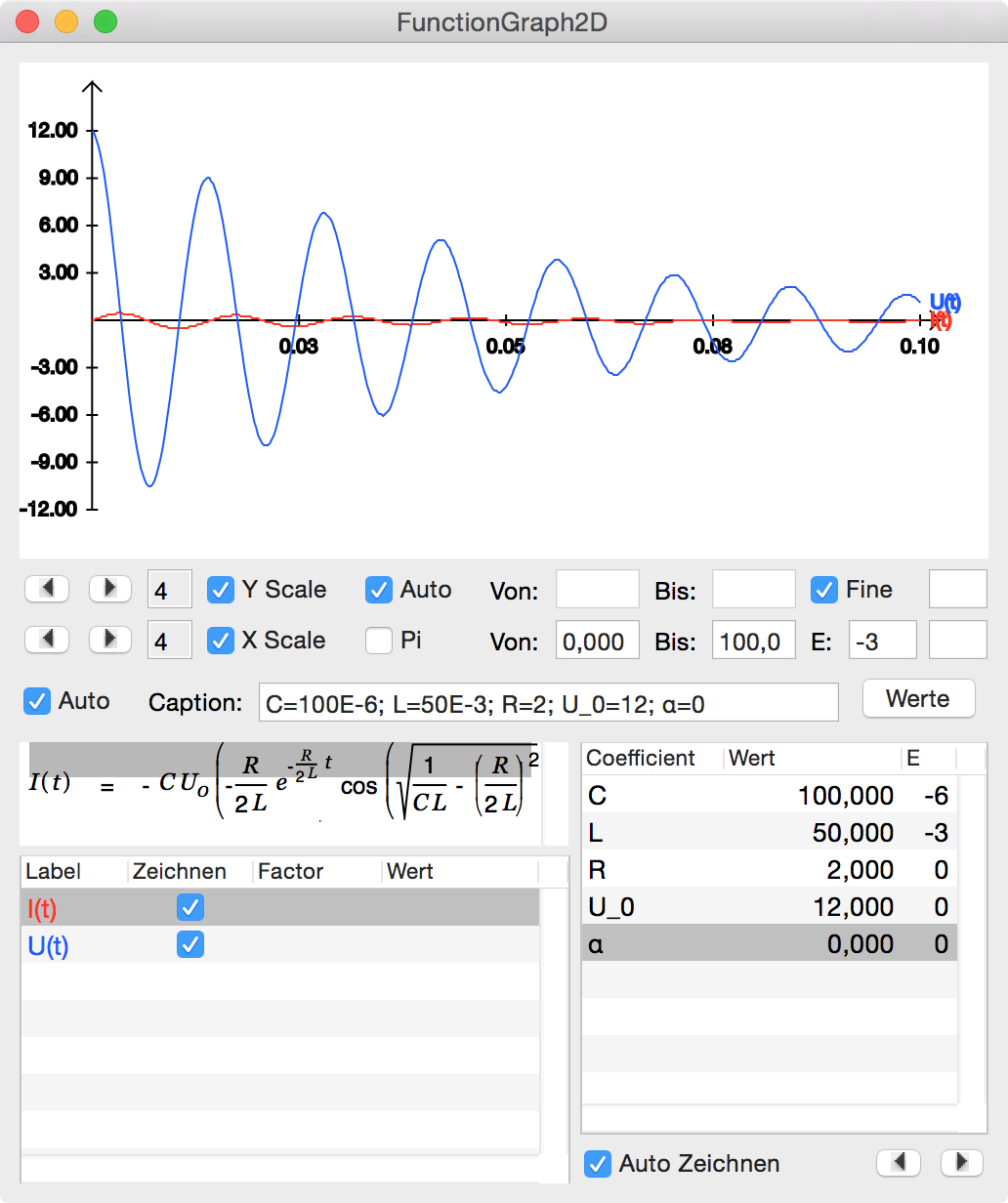This does not look too bad. However, the current line is too flat to be easily examined. We therefore specify a scale factor of ten for the current function.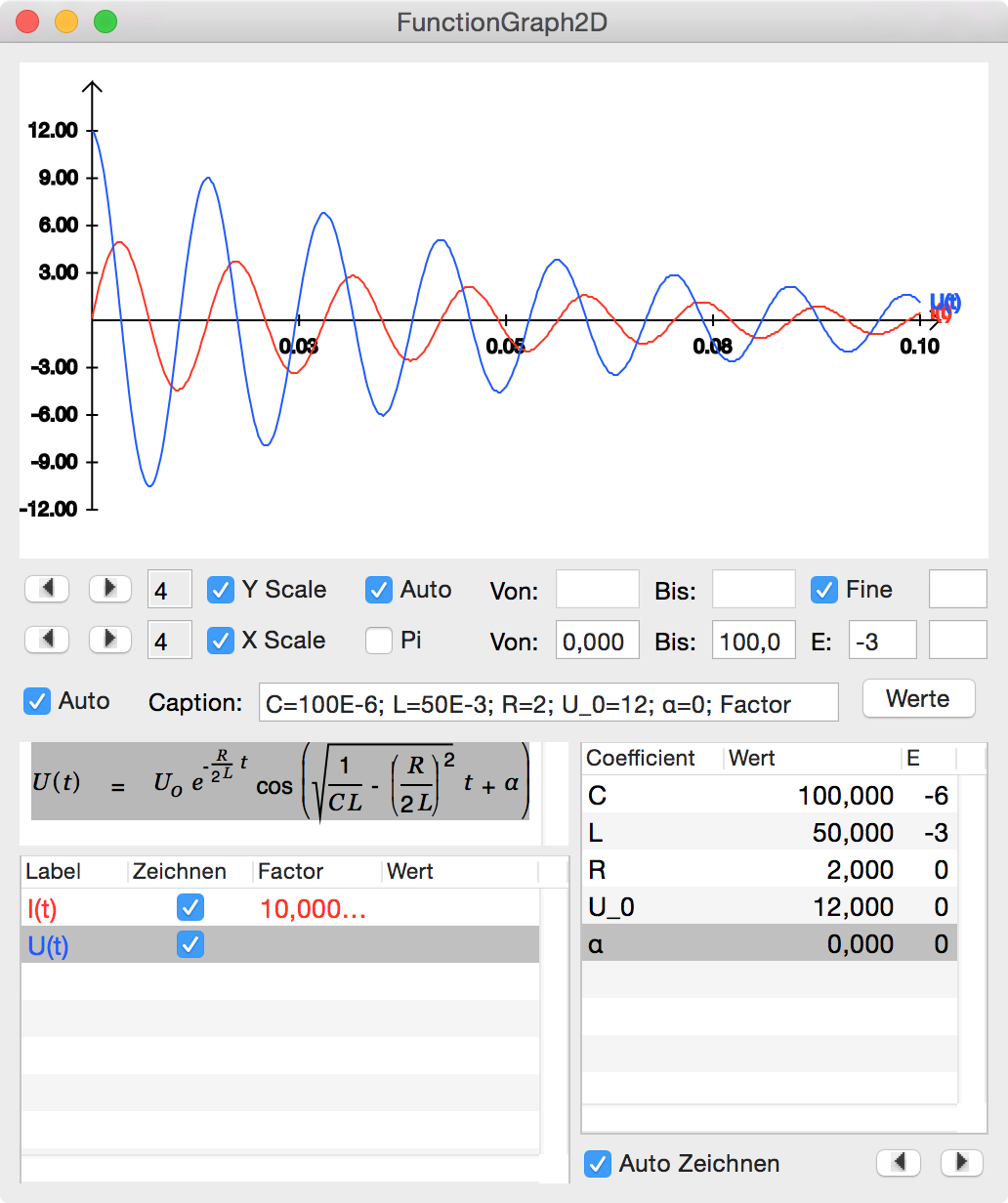Click back into your document. The graph is updated accordingly.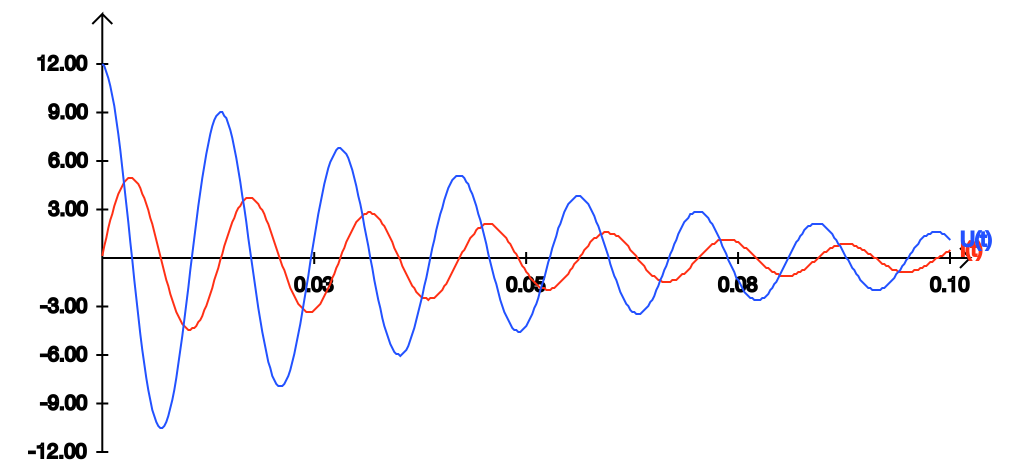We are not done yet. Let's assume we are interested in the heat loss in the wire resistance. This loss is given byWe Alt-drag the expression for the current onto this equation and getWe now double-click onto the 2D graph to (re)open its inspector and simply drag this last equation onto the textview. We also drag the expression for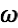onto the textview and set a scaler of 20 and a color for this additional physical property.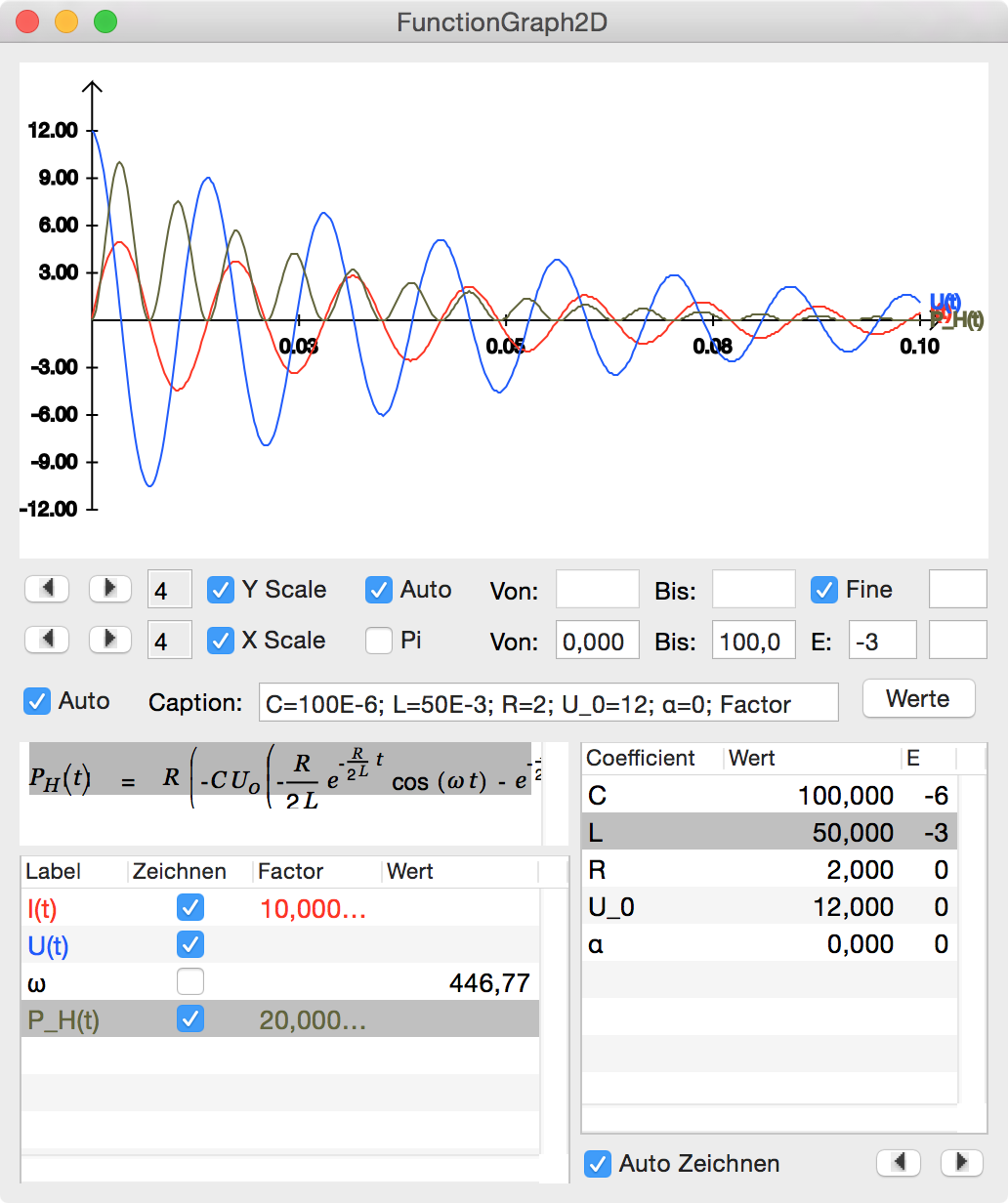Clicking back into the document updates the 2D graph.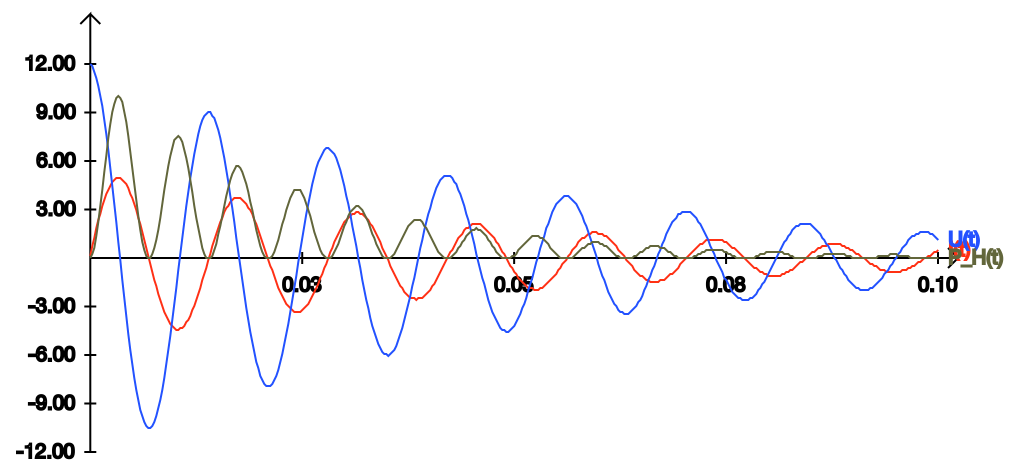The functions in a 2D graph can be interdependent. Assume the result of your creative work is the following set of functions (see Example Paper):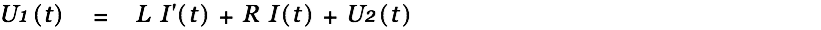Note that the last function depends on all the other. These functions can be dragged as they are onto a 2D graph to be plotted.## News

 23.04.2023 Cassiopeia 2.9.0 released 05.10.2022 Cassiopeia 2.8.3 released 29.09.2022 Cassiopeia 2.8.0 released 08.07.2022 Cassiopeia 2.7.0 released 14.04.2021 Cassiopeia 2.6.5 released 10.02.2021 Cassiopeia 2.6.1 released 26.06.2015 Word Processor Comparison 24.06.2015 Updated Documentation 23.06.2015 Cassiopeia Yahoo Group 18.06.2015 Advanced Data Security 11.05.2015 Cassiopeia Overview 08.05.2015 Exporting to files 14.05.2013 LaTeX and HTML Generation 08.05.2013 Example Paper released 26.04.2013 Co-editing in a workgroup 16.04.2013 Equation Editor Quick Reference 12.04.2013 Equation Editor 04.04.2013 Links and Bibliography 01.04.2013 Books 30.03.2013 Documents 28.03.2013 Simulations 16.03.2013 2D Graphs 10.03.2013 Symbolic Algebra 08.03.2013 Getting Started 07.03.2013 Installation and Setup

## White Papers

 13.10.2015 01 Writing documents 15.10.2015 02 Using the equation editor